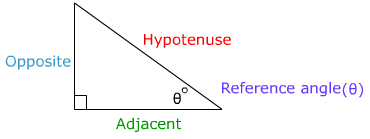# Introduction to the Six Trigonometric Functions (Ratios)

In right triangle Trigonometry there are six possible ratios (functions). A ratio is a comparison of two numbers (or sides of a triangle) by division. The Greek letter, θ, will be used to represent the reference angle in the right triangle.Opposite refers to the side of the triangle that is opposite of the reference angle

Adjacent refers to the side of the triangle that is adjacent to the reference angle.(the adjacent side will always form one side of the reference angle.

The hypotenuse is the side of the triangle that is always opposite the right angle.These six ratios represent all the ways to compare two sides of a right triangle. Notice that cosecant is the reciprocal of sine, secant is the reciprocal of cosine and cotangent is the reciprocal of tangent. The hypotenuse will never vary in its location however, the opposite and adjacent side will be determined by the reference angle.

 Related Links: Math Trigonometry SOH CAH TOA

To link to this Introduction to the Six Trigonometric Functions (Ratios) page, copy the following code to your site: